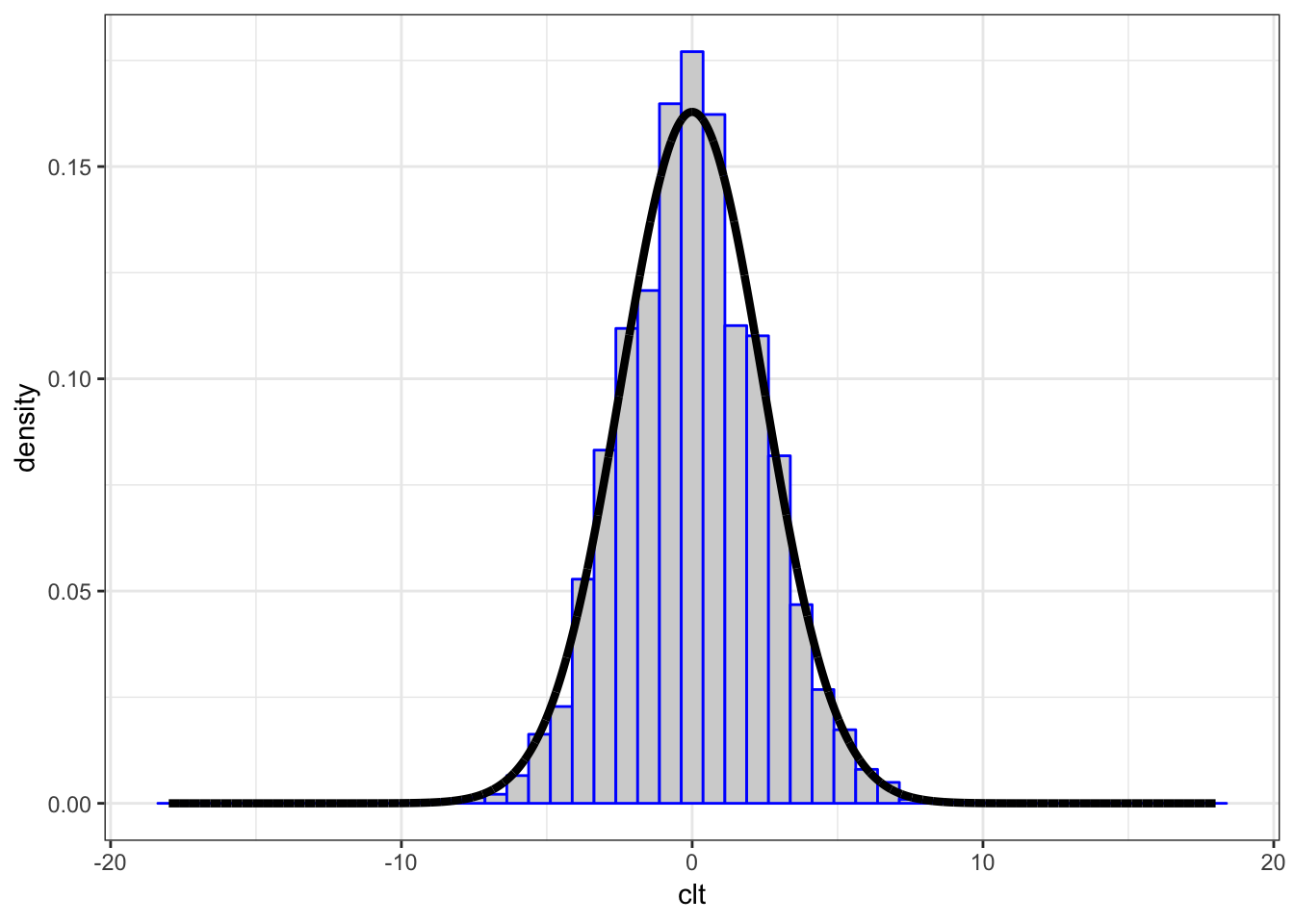18 Convergence of Random Variables

18.1 Sequence of RVs

Let $$Z_1, Z_2, \ldots$$ be an infinite sequence of rv’s.

An important example is

$Z_n = \overline{X}_n = \frac{\sum_{i=1}^n X_i}{n}.$

It is useful to be able to determine a limiting value or distribution of $$\{Z_i\}$$.

18.2 Convergence in Distribution

$$\{Z_i\}$$ converges in distribution to $$Z$$, written

$Z_n \stackrel{D}{\longrightarrow} Z$

if

$F_{Z_n}(y) = \Pr(Z_n \leq y) \rightarrow \Pr(Z \leq y) = F_{Z}(y)$

as $$n \rightarrow \infty$$ for all $$y \in \mathbb{R}$$.

18.3 Convergence in Probability

$$\{Z_i\}$$ converges in probability to $$Z$$, written

$Z_n \stackrel{P}{\longrightarrow} Z$

if

$\Pr(|Z_n - Z| \leq \epsilon) \rightarrow 1$

as $$n \rightarrow \infty$$ for all $$\epsilon > 0$$.

Note that it may also be the case that $$Z_n \stackrel{P}{\longrightarrow} \theta$$ for a fixed, nonrandom value $$\theta$$.

18.4 Almost Sure Convergence

$$\{Z_i\}$$ converges almost surely (or “with probability 1”) to $$Z$$, written

$Z_n \stackrel{a.s.}{\longrightarrow} Z$

if

$\Pr\left(\{\omega: |Z_n(\omega) - Z(\omega)| \stackrel{n \rightarrow \infty}{\longrightarrow} 0 \}\right) = 1.$

Note that it may also be the case that $$Z_n \stackrel{a.s.}{\longrightarrow} \theta$$ for a fixed, nonrandom value $$\theta$$.

18.5 Strong Law of Large Numbers

Suppose $$X_1, X_2, \ldots, X_n$$ are iid rv’s with population mean $${\operatorname{E}}[X_i] = \mu$$ where $${\operatorname{E}}[|X_i|] < \infty$$. Then

$\overline{X}_n \stackrel{a.s.}{\longrightarrow} \mu.$

18.6 Central Limit Theorem

Suppose $$X_1, X_2, \ldots, X_n$$ are iid rv’s with population mean $${\operatorname{E}}[X_i] = \mu$$ and variance $${\operatorname{Var}}(X_i) = \sigma^2$$. Then as $$n \rightarrow \infty$$,

$\sqrt{n}(\overline{X}_n - \mu) \stackrel{D}{\longrightarrow} \mbox{Normal}(0, \sigma^2).$

We can also get convergence to a $$\mbox{Normal}(0, 1)$$ by dividing by the standard deviation, $$\sigma$$:

$\frac{\overline{X}_n - \mu}{\sigma/\sqrt{n}} \stackrel{D}{\longrightarrow} \mbox{Normal}(0, 1).$

We write the second convergence result as above rather than $\frac{\sqrt{n} (\overline{X}_n - \mu)}{\sigma} \stackrel{D}{\longrightarrow} \mbox{Normal}(0, 1)$ because $$\sigma/\sqrt{n}$$ is the “standard error” of $$\overline{X}_n$$ when $$\overline{X}_n$$ is treated as an estimator, so $$\sigma/\sqrt{n}$$ is kept intact.

Note that for fixed $$n$$, ${\operatorname{E}}\left[ \frac{\overline{X}_n - \mu}{1/\sqrt{n}} \right] = 0 \mbox{ and } {\operatorname{Var}}\left[ \frac{\overline{X}_n - \mu}{1/\sqrt{n}} \right] = \sigma^2,$

${\operatorname{E}}\left[ \frac{\overline{X}_n - \mu}{\sigma/\sqrt{n}} \right] = 0 \mbox{ and } {\operatorname{Var}}\left[ \frac{\overline{X}_n - \mu}{\sigma/\sqrt{n}} \right] = 1.$

18.7 Example: Calculations

Let $$X_1, X_2, \ldots, X_{40}$$ be iid Poisson($$\lambda$$) with $$\lambda=6$$.

We will form $$\sqrt{40}(\overline{X} - 6)$$ over 10,000 realizations and compare their distribution to a Normal(0, 6) distribution.

> x <- replicate(n=1e4, expr=rpois(n=40, lambda=6),
+                simplify="matrix")
> x_bar <- apply(x, 2, mean)
> clt <- sqrt(40)*(x_bar - 6)
>
> df <- data.frame(clt=clt, x = seq(-18,18,length.out=1e4),
+                  y = dnorm(seq(-18,18,length.out=1e4),
+                            sd=sqrt(6)))

18.8 Example: Plot

> ggplot(data=df) +
+   geom_histogram(aes(x=clt, y=..density..), color="blue",
+                  fill="lightgray", binwidth=0.75) +
+   geom_line(aes(x=x, y=y), size=1.5)